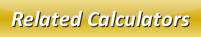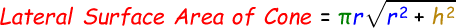# Lateral Surface Area of a Cone Calculator

The lateral surface area of a cone is the sum of surface area of all its overall face excluding cone's base.

We can find the lateral surface area of a cone with the help of this below formula:where,
r = radius of the cone
h = height of the cone

Note: Same formula is applied to calculate the surface area of a right cone.

In the below online lateral surface area of a cone calculator, enter the radius and the height of the cone and then click calculate to find the output.

 Radius of the Cone: Height of the Cone: Lateral Surface Area of Cone:

Latest Calculator Release

Average Acceleration Calculator

Average acceleration is the object's change in speed for a specific given time period. ...

Free Fall Calculator

When an object falls into the ground due to planet's own gravitational force is known a...

Torque Calculator

Torque is nothing but a rotational force. In other words, the amount of force applied t...

Average Force Calculator

Average force can be explained as the amount of force exerted by the body moving at giv...

Angular Displacement Calculator

Angular displacement is the angle at which an object moves on a circular path. It is de...